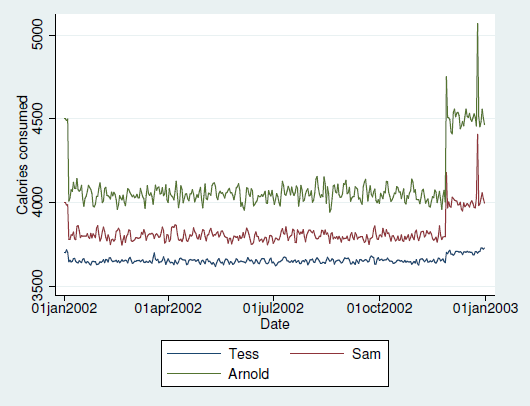»  Home »  Products »  Training »  NetCourses »  NetCourseNow »  NCNow471: Introduction to panel data using StataTraining

## NetCourseNow™ 471: Introduction to panel data using Stata

\$495

Discounts available for enrollments of
two or more participants.

NetCourseNow is a self-paced, personalized learning experience. All lessons will be posted at once, and you will be given the email address of your personal NetCourse instructor, to whom you can email questions about the lessons. Enroll now, and begin when you're ready.

Content:
Become an expert in the analysis and implementation of linear, nonlinear, and dynamic panel-data estimators using Stata. This course focuses on the interpretation of panel-data estimates and the assumptions underlying the models that give rise to them. The course is geared for researchers and practitioners in all fields. The breadth of the lectures will be helpful if you want to learn about panel-data analysis or if you are familiar with the subjects.

Prerequisites:

• Stata 17 or Stata 16, installed and working
• Course content of NetCourse 101 or equivalent knowledge
• Familiarity with basic time-series, cross-sectional summary statistics and linear regression
• Internet web browser, installed and working
(course is platform independent)

#### Course content

Lesson 1

• An introduction to panel data and its features
• Getting started with panel data
• Summary statistics and dynamics
• Overview of basic concepts
• Data generation
• The regression model
• Variance-covariance estimators
• Margins and marginal effects
• Basic panel-data estimation concepts
• Moment-based estimation
• Panel data, regression, and efficiency
• Closing remarks

Lesson 2

• Random-effects model
• The model
• Fixed-effects model
• Within estimator
• Comparing within and random-effects estimates
• First-differenced estimator
• Deciding between random and fixed effects
• Hausman test
• Mundlak test
• Population-averaged models

Lesson 3

• Probit model
• Probit models for panel data: Random effects
• Probit models for panel data: Population averaged
• Probit models for panel data: Remarks
• Logit model
• Logit models for panel data: Random Effects
• Logit models for panel data: Fixed Effects
• Logit models for panel data: Population Averaged
• Poisson model
• Poisson models for panel data

Lesson 4

• Endogeneity
• Cross-sectional estimation under endogeneity
• Panel-data estimation under endogeneity
• Dynamic models
• Building your own dynamic models
• A more complex dynamic structure
• Concluding remarks